# Troops

If the general sorts troops into the crowd by nine left 6. How many soldiers has regiment if we know that they are less than 300?

Correct result:

n =  294

#### Solution:

$300/9 = 33.333333333333 \ \\ 297 = 33\cdot 9 \ \\ 288= 32\cdot 8 \ \\ n = 32\cdot 8 +6 = 294$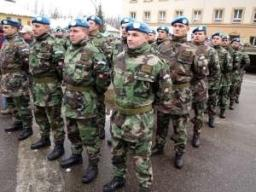We would be pleased if you find an error in the word problem, spelling mistakes, or inaccuracies and send it to us. Thank you!## Next similar math problems:

• Decomposition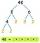Make decomposition using prime numbers of number 155. Result write as prime factors (all, even multiple)
• Troops - soldiers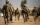Food stocks will last 20 soldiers of the 4-day training. How many days they could extend training if four soldiers become sick?
• Cash back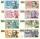After a trip 24 students have left more than 650 but less than 690 CZK (Kc). How much each student receives return when the amount is divisible by the number of pupils?
• TroopsThe route is long 147 km and the first-day first regiment went at an average speed of 12 km/h and journey back 21 km/h. The second day went second regiment the same route at an average speed of 22 km/h there and back. Which regiment will take route longer
• School booksAt the beginning of the school year, the teacher distributed 480 workbooks and 220 textbooks. How many pupils could have the most in the classroom?
• Trees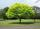Loggers wanted to seed more than 700 and less than 800 trees. If they seed in rows of 37, left them 8 trees. If they seed in rows of 43, left the 11 trees. How many trees must seed ?
• Dressmaker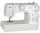Dressmaker left a piece of canvas shorter than 5 meters. She is deciding whether it sew a skirt or dress. The canvas was exactly as much as they consumed had cut up her skirt to 120 cm, or 180 centimeters dress. What a piece of canvas left her?
• Game roomWinner can took win in three types of jettons with value 3, 30 and 100 dollars. What is minimal value of win payable in this values of jettons?
• PlumsIn the bowl are plums. How many would be there if we can divide it equally among 8, 10 and 11 children?
• TilesHall has dimensions 325 &time; 170 dm. What is the largest size of square tiles that can be entire hall tiled and how many we need them?
• PegsFrom two sticks 240 cm and 210 cm long, it is necessary to cut the longest possible pegs for flowers so that no residues remain. How many pegs will it be?
• Prime divisorsFind 2/3 of the ratio of the sum and the product of all prime divisors of the number 120.
• Gardens colonyGardens colony with dimensions of 180 m and 300 m are to be completely divided into the same large squares of the highest area. Calculate how many such squares can be obtained and determine the length of the square side.
• Tram stop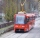The blue tram stops every 12 minutes, the red one 8 minutes. At 8 o'clock they left the stop together. How many times do they meet at a stop before 11 am?
• Count of roots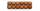How many solutions has equation x. y = 7757 with two unknowns on the set of natural numbers?
• Matches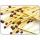George poured out of the box matches and composing them triangles and no match was left. Then he tries squares, hexagons and octagons and no match was left. How many matches must be at least in the box?
• DivisibilityDetermine all divisors of number 84.